# Q-Intro: Amplitude Amplifier

In the previous introductory article, we have discussed about the Inversion about the mean and quantum circuit along with derivation.

In this present introduction article, we discuss about the amplitude amplification using phase inversion/phase kick-back and inversion about the mean.

To get an overview about Phase kick back or phase inversion, let’s recall Deutsch-Jozsa Algorithm. Below is the circuit for n qubit case. which follows a superposition, manipulate the state and finally collapse superposition by measuring.

at the initial stage as shown below,

after applying Hadamard gates, let H|0⟩ = |x⟩ and we know H|1⟩ = |-⟩ as shown below

after applying Oracle Uf,

and further simplifying such as f(x) = 1 when x equals to x0 and f(x) = 0 when x not equals to x0 taking out common’s as shown below

Let’s explore further by considering an example, n=2

How does this unitary operation act on states?

If |x⟩ be in a equal superposition of four different states as shown below

the state vector looks as shown below

when x = x0 then at |ψ2⟩ phase change happens incase of x0 as -1,

by choosing x0 = “10” (numeric : 2) and performing a phase inversion, the state looks like

Performing Measurement on |x⟩ does not give any information as the amplitude is squared as shown below,

Changing the phase from positive to negative separates the phases, but after measurement does not separate them enough.

How can we separate them enough to differentiate at the measurement?

Well, What we needed is a way of boosting the phase separation of the desired binary string from the other binary strings.

The second trick is called inversion about the mean (refer previous article) or inversion about the average. This is a way of boosting the separation of the phases.

Example: Let’s see how both these techniques(phase inversion and inversion about the mean) work together.

let’s consider a vector “v” as shown below

phase inversion means introducing negative sign before the selected element which we have observed from the above algorithm. Let select the element 3 from above 5 elements vector and introduce negative sign as shown below

The average of these 5 numbers is a = (20+20–20+20+20)/5 = 60/5 = 12.

Calculating the inversion about the mean we get

in case of element 20: −v + 2a = −20 + (2 × 12) = 4 and

in case of element -20: −v + 2a = 20 + (2 × 12) = 44

Thus, our five numbers become

The difference between the third element and all the others is 44 − 4= 40.

Great! we are able to create a difference in amplitude specially the chosen one which is 3rd element out of 5 elements.

Quantum Circuit:

combining both the circuits of phase inversion and inversion about the mean as follows

Example: Let us look at an example of an execution of this algorithm. Let f be a function for n =4 that picks out the string “1101”

points 1 and 2 in the above circuit are at the top register only where as 3 and 4 for both registers. The states after each step will be

At point 1 as shown below

At point 2 as shown below

At point 3, after applying oracle to the above wave function the phase inversion shall happen as shown below

The average of the numbers mentioned in the above wave function is

Calculating the inversion about the mean or inversion about the average as discussed in the previous article, we get

in case of element -1/4 : −v + 2a

in case of element 1/4 : −v + 2a

At point 4 after performing the inversion about mean we have state as shown below

calculating 3/16 = 0.1875 and 11/16 = 0.6875 (round off)

Squaring the numbers gives us the probability of measuring those numbers as 0.6875² = 0.4726 and 0.1875² = 0.0351 from this probability distribution we can easily differentiate the state we have chosen through quantumly and also sum of probabilities shall always be equal to 1

0.4726 + 15 * 0.0351 = 0.4726 + 0.5273 = 0.9999 due to round off

When we measure the state in Step 4, we will most likely get the state

if we take difference between the 14th element and all the others is 11/16 − 3/16 = 8/16 = 1/2 = 0.5

Shall we increase the difference between the 14th element and all others further more?

if we are able to increase the difference further the probability distribution for 14th element further increases, which further boosts the confidence value of the identifier.

Let’s repeat the phase inversion and inversion about mean over the previous values.

After performing the phase inversion

the average of the elements mentioned in the above vector as follows

Calculating the inversion about the mean or inversion about the average as discussed in the previous article, we get

in case of element -11/16 : −v + 2a

in case of element 3/16 : −v + 2a

after performing the inversion about mean we have state as shown below

calculating 5/64 = 0.0781 and 61/64 = 0.9531 (round off)

if we take difference between the 14th element and all the others is 61/64 − 5/64 = 56/64 = 7/8 = 0.875

Squaring the numbers gives us the probability of measuring those numbers as 0.9531² = 0.9083 and 0.0781² = 0.0060

Comparatively, We have further separated the numbers. 1st time difference is 0.5 and second time difference is 0.875

Will the numbers be separated further, if we try this iteration one more time(applying phase inversion and inversion about the mean)?

Let’s repeat the phase inversion and inversion about mean over the previous values.

After performing the phase inversion

the average of the elements mentioned in the above vector as follows

Calculating the inversion about the mean or inversion about the average as discussed in the previous article, we get

in case of element -61/64 : −v + 2a

in case of element 5/64 : −v + 2a

after performing the inversion about mean we have state as shown below

calculating -13/256 = -0.0507 and 251/256 = 0.9804 (round off)

if we take difference between the 14th element and all the others is 251/256 + 13/256 = 264/256 = 1.0312

Squaring the numbers gives us the probability of measuring those numbers as 0.9804² = 0.9611 and -0.0507² = 0.0025

Comparatively, We have further separated the numbers, which indeed increases the probability of finding from 0.9083 to 0.9611

This is going really interesting let’s try one more time(applying phase inversion and inversion about the mean)!

Let’s repeat the phase inversion and inversion about mean over the previous values.

After performing the phase inversion

the average of the elements mentioned in the above vector as follows

Calculating the inversion about the mean or inversion about the average as discussed in the previous article, we get

in case of element -251/256 : −v + 2a

in case of element -13/256 : −v + 2a

after performing the inversion about mean we have state as shown below

calculating -153/512 = -0.2988 and 323/512 = 0.6308 (round off)

if we take difference between the 14th element and all the others is 153/512 + 323/512 = 476/512 = 0.9296

Squaring the numbers gives us the probability of measuring those numbers as -0.2988² = 0.0892 and 0.6308² = 0.3979

Comparatively, We have not further separated the numbers and also the probability of finding reduced from 0.9611 to 0.3979

This is really interesting!, Will it further reduce if we perform the iteration one more time?

Let’s repeat the phase inversion and inversion about mean over the previous values.

After performing the phase inversion

the average of the elements mentioned in the above vector as follows

Calculating the inversion about the mean or inversion about the average as discussed in the previous article, we get

in case of element -323/512 : −v + 2a

in case of element -153/512 : −v + 2a

calculating -17/2048 = -0.0083 and -697/2048 = -0.3403 (round off)

if we take difference between the 14th element and all the others is 697/2048 -17/2048 = 680/2048 = 0.3320

Squaring the numbers gives us the probability of measuring those numbers as -0.0083² = 0.00006 and -0.3403² = 0.1158

Comparatively, We have not further separated the numbers and also the probability of finding reduced from 0.3979 to 0.1158

Observations:

1. First Observation:

In our example we have considered the superposition with Hadamard gate, but in the case of amplitude amplification it is not always necessary that we shall keep qubits in superposition with the Help of Hadamard gates only. We can use any Unitary Gate to create a rotation other than Hadamard rotation.

2. Second Observation:

from the above table we can see that after iteration 3 onwards the probability value gets decrease.

This raises a question in our mind In General, what shall be the threshold value such that we get high probability value to observe the required result.

References: qiskit textbook, cirq tutorials, IITM course by (@Prabha M, Anil Prabhakar), Qubit by Qubit(The Coding School) course, Textbook by Noson S, Wikipedia, stack exchange and open source materials.

--

--

--

## More from Anonymousket

Love podcasts or audiobooks? Learn on the go with our new app.

## The least informative pulse## Researching the Mathematics Behind the Stellar Consensus Protocol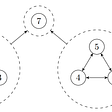## Algorithms of Creation## The Virtue of Causal Inference — Part 2 : Notation, Assumption, and Bias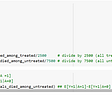## The Existence of Luck Explained using Brownian Motion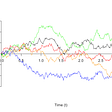## TK Computing Area Using Calculus## Unsolvable Problems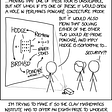## How I Finally Understood the Famous Monty Hall Problem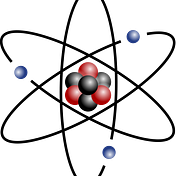## Single-Photon Sources (Part 2)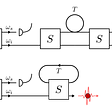## Analytical gradient of energies with a sophisticated calculation method by quantum computers…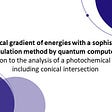## Quantum Key Distribution